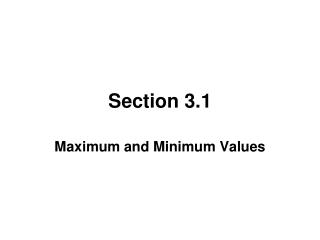# Section 3.1 - PowerPoint PPT PresentationDownload PresentationSection 3.1

Section 3.1Download Presentation## Section 3.1

- - - - - - - - - - - - - - - - - - - - - - - - - - - E N D - - - - - - - - - - - - - - - - - - - - - - - - - - -
##### Presentation Transcript

1. Section 3.1 Maximum and Minimum Values

2. ABSOLUTE MINIMUM AND ABSOLUTE MAXIMUM • A function f has an absolute(or global) maximum at c if f(c) ≥ f (x) for all x in D, where D is the domain of f. The number f (c) is called the maximum value of f on D. • A function f has an absolute (or global) minimum at c if f(c) ≤ f (x) for all x in D, where D is the domain of f. The number f (c) is called the minimum value of f on D. • The maximum and minimum values of f are called the extreme values of f.

3. LOCAL MINIMUM ANDLOCAL MAXIMUM • A function f has a local (or relative) maximum at c if f (c) ≥ f (x) for all x near c. • A function f has a local (or relative) minimum at c if f (c) ≤ f (x) for all x near c.

4. THE EXTREME VALUE THEOREM Theorem: If f is continuous on a closed interval [a, b], then f attains an absolute maximum value f (c) and an absolute minimum value f (d) at some numbers c and d in [a, b].

5. FERMAT’S THEOREM Theorem: If f has a local maximum or minimum at c, and if f′(c) exists, then f ′(c) = 0.

6. CRITICAL NUMBERS A critical number of a function f is a number c in the domain of f such that either f ′(c) = 0 or f′(c) does not exist.

7. FERMAT’S THEOREM REVISITED Fermat’s Theorem can be stated using the idea of critical numbers as follows. Fermat’s Theorem: If f has a local minimum or local maximum at c, then c is a critical number of f.

8. THEOREM If f is continuous on a closed interval [a, b], then f attains both an absolute minimum and absolute maximum value on that interval.

9. THE CLOSED INTERVAL METHOD To find the absolute maximum and minimum values of a continuous function on a closed interval [a, b]: • Find the values of f at the critical numbers of f in (a, b). • Find the values of f at the endpoints of the interval. • The largest of the values from Steps 1 and 2 is the absolute maximum value; the smallest of these values is the absolute minimum value.